# 使用.NET语言开发Silverlight应用程序入门（三）：画刷和变形

A. 画刷（Brush）

1. SolidColorBrush< Rectangle  Width ="200"  Height ="150"   >< Rectangle .Fill >< SolidColorBrush  Color ="Black"   /></ Rectangle.Fill ></ Rectangle >< Rectangle  Width ="200"  Height ="150"  Fill ="Black"   />< Rectangle  Width ="200"  Height ="150" >< Rectangle .Fill >< LinearGradientBrush  StartPoint ="0,0"  EndPoint ="1,1" >< GradientStop  Color =“Blue"  Offset ="0"   />< GradientStop  Color ="Black"  Offset ="1"   /></ Rectangle.Fill ></ Rectangle >< Rectangle  Width ="200"  Height ="150"   >< Rectangle .Fill >< GradientStop  Color ="Red"  Offset ="0"   />< GradientStop  Color ="Black"  Offset ="1"   /></ Rectangle.Fill ></ Rectangle >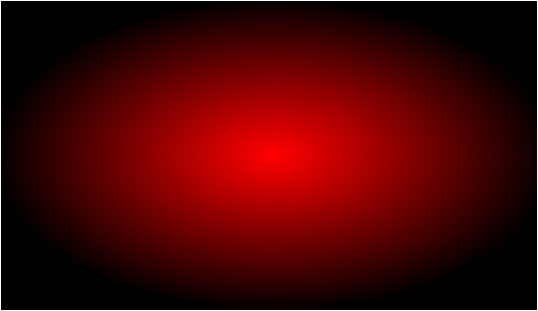3. ImageBrush< Ellipse  Width ="200"  Height ="75"   >< Ellipse .Fill >< ImageBrushImageSource ="http://.../XBox360Logo.jpg"   /></ Ellipse.Fill ></ Ellipse >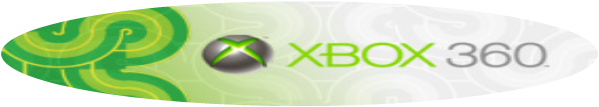4. VideoBrush< MediaElement  x:Name =“MyVideo”  Source =“Video.wmv”  IsMuted =“true”/><Ellipse Width ="200"  Height ="75"   >< Ellipse .Fill >< VideoBrush  SourceName =“MyVideo”/></Ellipse.Fill ></ Ellipse >

B. 变形（Transform）

Silverlight里几乎所有的元素都支持变形操作。常见的变形操作有如下几个。为了更好的说明每一类的变形效果，请先记住下面这张脸：）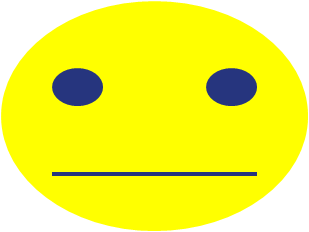• 旋转变形：<RotateTransform/>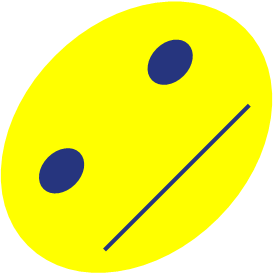• 缩放变形：<ScaleTransform/>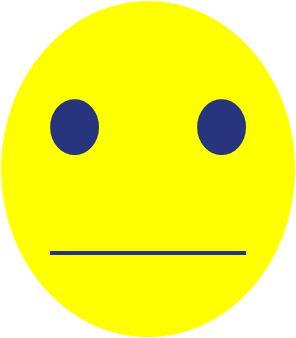• 扭曲变形：<SkewTransform/>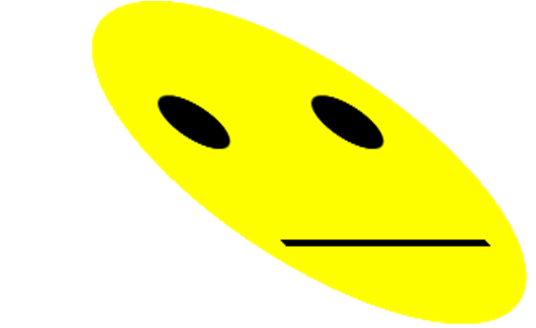• 移动变形：<TranslateTransform/>

• 组合变形：<MatrixTransform/>< TextBlock  Text ="Hello World" >< TextBlock .RenderTransform >< RotateTransform  Angle ="45"   /></ TextBlock.RenderTransform ></ TextBlock >< Canvas  x:Name ="Content"  Width ="500"  Height ="500" >< TextBlock  FontSize ="24“>Button still works!</TextBlock><mybutton:Button Text="
Push Me" Canvas.Top ="50"   />< Canvas .RenderTransform >< TransformGroup >< RotateTransform  Angle ="45" />< SkewTransform  AngleX ="45" /></ TransformGroup ></ Canvas.RenderTransform ></ Canvas >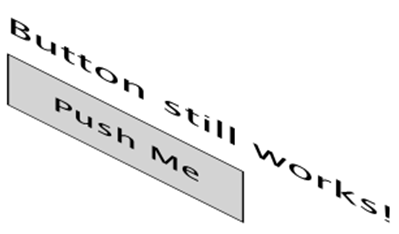09-238506
10-0950
10-0393
01-0235
09-0715
01-305655
10-3157
03-178447
09-2613万+